diff_months: 11

# Engineering Simulation -PDE4905 Assignment

Order Code: 483390
• Subject Code :

PDE4905

Task 1 Simple Linear Programming and Optimisation (20 Marks)
A small factory has two products (A and B). Each product A costs \$(80-a) to produce, and each product B costs \$(60-b) to produce (where a and b are the last digits of your student number, respectively). The total costs must be less than \$5000 as the budget each day. In addition, the market regulations require that the number of product B cannot exceed twice the number of product A, subject to the extra requirement that the number of each product must be under 50 units per day.Each product A gives a profit of \$40 and each product B gives a profit of \$60. Design a simple daily production portfolio (in terms of the numbers of Products A and B to be produced) so that the total profit is maximized.
• Write this problem as a simple linear programming problem with the objective and all appropriate constraints.
• Find its optimal solution by solving it using two different methods (e.g., graphical method and Excel Solver/Matlab).
• If the factory wants to increase its profit by 20%, please advise what should be done to achieve this?[Hint. You can try to increase the budget, if so, by how much?]
• If you cannot obtain integer solutions, please briefly discuss the reason and how to modify the formulate so that you can obtain integer solutions (the numbers of units of both products must be integers). If you can obtain the integer solutions in the first place, also explain why it is the case.

Task 2 Choose one from two options (either 2a or 2b)
(2a) Transportation/Resource Allocation [25 Marks]
A product has 4 suppliers (A, B, C, D) that produce a total of 2000 units. All the units produced should be distributed to 6 demand stations/customers (P, Q, R, S, T, W). The ransportation costs for transporting one unit from a supplier to a station are given in the following table: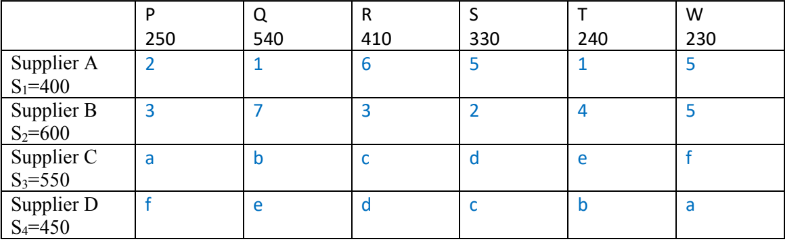Thus, its transportation cost matrix is simply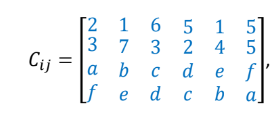where a, b, c, d, e, and f are the single digits of your student number in the format “M00abcdef”. For
example, if your number is M00152379, you have a=1, b=5, c=2, d=3, e=7 and f=9.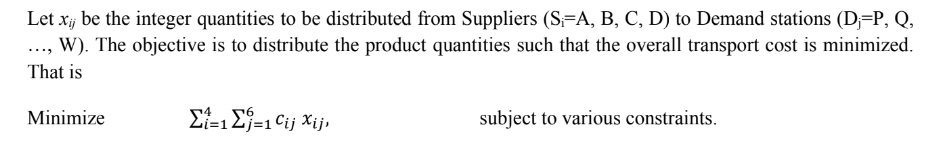• Write down the mathematical formulation for this problem with the objective and all relevant constraints and discuss their meaning briefly.
• Solve the problem using either Excel Solver or Matlab/Octave to find its optimal solution.• Check if the solution can indeed satisfy all the constraints.

(2b) Supermarket Staff Scheduling (25 Marks)
A busy supermarket is open 24 hours daily. It has a set of 20 checkout counters, though the actual number of checkouts needed ranges from 3 to 20, depending on the time of day. The number of staff needed to provide a satisfactory service varies at different hours as summarized in the following Table: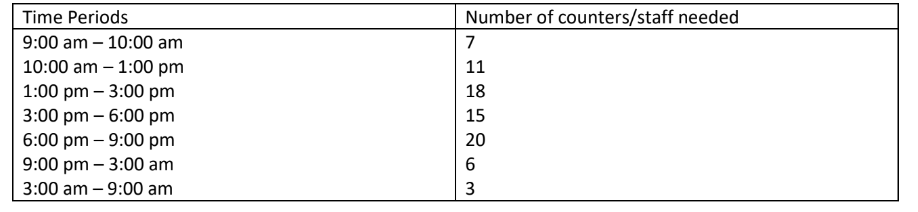The supermarket employs 15 full-time staff and a large number of part-time staff. Part-time staff typically can work a 6-hour shift every day and can start on the hour between 9:00 am to 1:00 pm, or at 3:00 pm, 6:00 pm,9:00 pm or 3:00 am.
Full-time staff work at most 40 hours per week between 9:00 am to 5:00 pm with a daily wage of £110 for 8 hours per day, while part-time workers are paid at a standard rate of £10 per hour (i.e., £60 per day for 6 hours).The company policy only permits that up to 75% of the total working hours of any day to be part-time hours.The main task is to schedule the staff so that the total staff costs are minimized on a daily basis.
• Formulate the scheduling problem in terms of a linear programming problem with a correct objective and all appropriate constraints. Explain in detail why your mathematical formulation is appropriate,
including any assumptions that you may have used.

• Solve your staff scheduling problem using either Excel Solver or Matlab (intlinprog)/Octave (glpk).Summarize your solution procedure (including screenshots if appropriate) and present the main results with a brief discussion.
• Check if all the required numbers of counters are met.

Task 3 Manufacturing Portfolio (30 Marks)
An engineering tooling manufacturer has 4 products: machining tables, tooling sets, testing tools, and storage boxes. A machining table takes 8 hours to produce with the material cost of £80. A tooling set takes 6 hours to make with the material cost of £20. A testing tool takes 4 hours to make with the material cost of £25. A storage box takes 2 hours to make with the material cost of £10. Each week,there are 800 hours available with a weekly budget of £4000 for material costs. To make maintain diversity of the products, the company will produce at least 5 units for each product.
A machining table typically comes with a tooling set. Thus, the number of tooling sets should be at least 3 times the total number of machining tables and testing tools. Some customers may also wish to buy a storage box if they buy a tooling set. Thus, the number of storage boxes should not exceed the number of tooling sets.The profits per unit are £250, £100, £150, and £60 for machining tables, tooling sets, testing tools,storage boxes, respectively. The main objective is to organize the production portfolio so as to
maximize the profit of the company on a weekly basis.
a) Formulate this problem as an LP with the objective and all the appropriate constraints.b) Solve the LP and find the maximum profit and discuss your result.
c) The company seems to do well, so it decides to hire more people (thus more available hours)with the same material cost budget. If the number of available hours is now 1000, will it affect the production portfolio? Should the company recruit more people? Discuss and explain.
d) Another strategy is to increase the budget for raw materials. If the company intends to increase its profit by 15%, subject to the condition that the available number of hours remains unchanged, what is the new budget for raw material? Will the increase in the budget of raw
e) The company later realizes that the testing tools do not sell well, so it may consider not to produce any testing tools at all. Will this potential change increase or decrease the profit
(assuming that the other conditions remain the same)? Discuss and explain briefly.

Based on the Simulink model for a single motor you have built in class, now we have two identical motors that are attached to two light bars as two arms to form a simple robotic arm model (see Fig. 1).
Both arms have the same length of 500 mm. The two arms can rotate independently, and you can control each motor individually with the same or different voltages.

For simplicity, you can use the same parameters that you have used in class, and you can also ignore the masses of the two light robot arms (thus no change in the moment of inertia).
• Build a Simulink model to simulate the robotic arm rotation. [Hint. You can use the model you have built in class as the basis for extending the model functions.]
• Rotate Arm 1 by 45o and rotate Arm 2 by 90o , change your model parameters (e.g., feedback and/or voltage) to achieve the desired rotation angles. Sketch the response curves.
• Discuss how to rotate Arm 1 by -90o and rotate Arm 2 by +90o ? What is the Cartesian location of point B (assuming the base of Motor 1 as the origin)? Show your response curves.
• Point A will move on a circle of radius R=500 mm. Discuss the shape or domain that point B can cover by moving both motors. If point B is constrained within a rectangular region from
[-500,250] to [+500, +250], is it possible to achieve this. If yes, how? If not, why not?Discuss briefly (no more than half a page).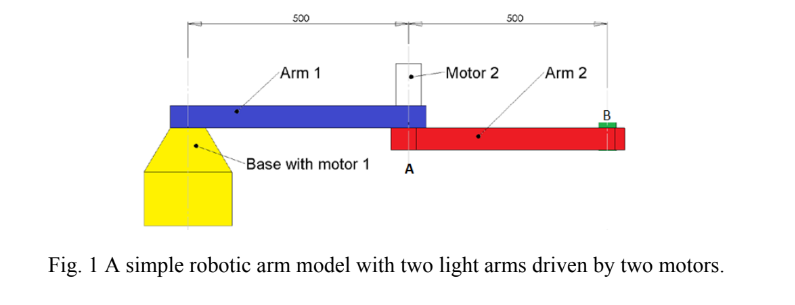• Uploaded By : Katthy Wills
• Posted on : January 11th, 2023
• Views : 95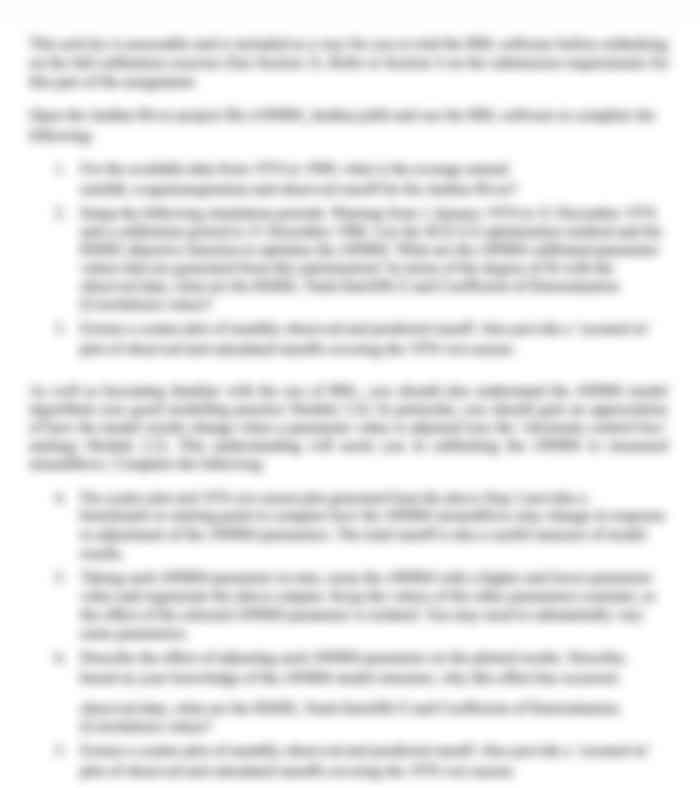## Choose a Plan

80 USD
• All in Gold, plus:
• 30-minute live one-to-one session with an expert
• Understanding Marking Rubric
• Structuring & Formatting
• Referencing & Citing
Most
Popular

### Gold

30 50 USD
• Get the Full Used Solution
(Solution is already submitted and 100% plagiarised.
Can only be used for reference purposes)
Save 33%

### Silver

20 USD
• Journals
• Peer-Reviewed Articles
• Books
• Various other Data Sources – ProQuest, Informit, Scopus, Academic Search Complete, EBSCO, Exerpta Medica Database, and more